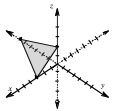### Home > A2C > Chapter 10 > Lesson 10.2.6 > Problem10-158

10-158.
1. Graph in three dimensions. Homework Help ✎

1. (2, −1, −3)

2. 6x − 3y + 9z = 18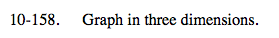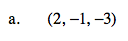Remember (x, y, z).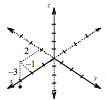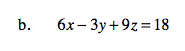Simplify the equation by dividing both sides by 3.

2xy + 3z = 6

Find the intercepts.